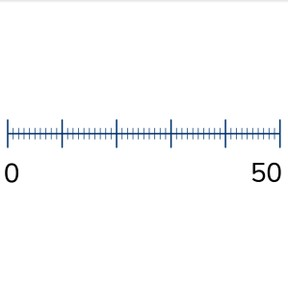Numbers to 50 on the number line

# Numbers to 50 on the number line

Numbers to 50 on the number line8,000 schools use Gynzy92,000 teachers use Gynzy1,600,000 students use Gynzy

## General

Students learn to place numbers from 0-50 on the number line. They learn to count forward and back and that every line represents a number.

K.CC.A.1
K.CC.A.2

## Relevance

Discuss with students that it is important to be able to place numbers on a number line, so they know where a number belongs. They can use this to determine when a number is more or less.

## Introduction

Ask students to work in pairs. Have them count to 50. The first student, who is counting out loud to 50 should skip a number every so often. The second student must clap their hands when the first skips a number and say what the missing number is. The first student continues counting until they reach 50 (with more skipped numbers). The students then swap and count to 50 again.

## Development

Explain what a number line is to students and tell them that number lines can look different. Tell them that a number line starts with the lowest number and that the numbers are in counting order. Show students a number line that only shows the tens numbers. Practice placing the tens numbers on the number line. Ask students to determine which number belongs at the arrow. Next show a number line with the tens numbers, but with shorter lines between the tens numbers. Say that these shorter lines represent one number each. Discuss with students that counting from left to right is counting forwards, and counting right to left is counting backwards. Look to see which tens number the arrow is closest to. Use that to determine if you should count forwards or backwards. Practice together with students with determining which number the arrow is pointing at and by dragging the numbers to their correct positions.

Check that students understand placing numbers to 50 on the number line by asking the following questions:
- What is a number line?
- How do you place a number on the number line?
- Where do you start counting from?
- When do you count forwards and when do you count back?

## Guided Practice

Students first practice placing numbers on a number line where they count forward or back on the number line. They practice on a number line with tens numbers filled in. Next they practice on part of a number line with tens numbers shown and finally they practice with a blank number line.

## Closing

Discuss with students that it is important to know how to place numbers on a number line, because then you know where a number belongs. Check that students know what a number line is and ask them to discuss in groups which numbers belong at the arrows.

## Teaching Tip

Students who have difficulty placing numbers on the number line to 50 can be supported by practicing counting forwards and backwards to 50, and by drawing a number line with all the numbers to 50.

### The online teaching platform for interactive whiteboards and displays in schools

• Save time building lessons

• Manage the classroom more efficiently

• Increase student engagement